NYQUIST STABILITY CRITERION

I. Setup and Assumptions:

Feedback System: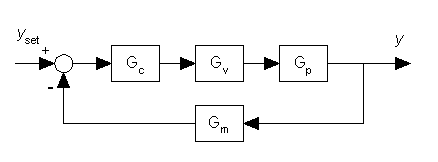Figure 1.

Closed Loop Transfer Function: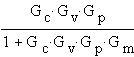Characteristic Equation:         1 + GcGvGpGm=0

(Note: This equation is not a polynomial but a ratio of polynomials)

Stability Condition: None of the zeros of ( 1 + GcGvGpGm )are in the right half plane.

Assumption: Gc, Gv, Gp and Gm are all stable, i.e. stable (open-loop) process, controller, actuator and sensor. This means (1 + GcGvGpGm ) has no poles in the right half plane.

II.  Objective : to determine the stability of a feedback control system using frequency response data.

III. Preliminaries:

a) A transfer function G(s) is said to be proper if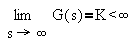and strictly proper if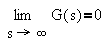(Note: All physically realizable systems are either proper or strictly proper.)
b) If G(s) is a ratio of polynomial functions, then
• G(s) is proper if the order of the numerator polynomial is less than or equal than the order of the denominator polynomial
• G(s) is strictly proper if the order of the numerator polynomial is less than the order of the denominator polynomial
c) Nyquist path:

In the s-plane, the clockwise path A-B-C-A is a simple closed semi-circle contour in the right half of the complex plane as shown below: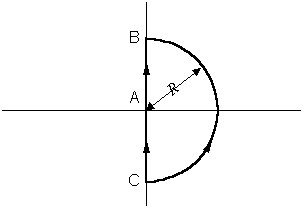Figure 2.

As R approaches infinity, the contour will encompass all the points in the right half plane.

d) Complex mapping using the Nyquist path:

Let G(s) be proper, then
-  The mapping of G(s) along the path A-B is just G(iw) for w=0 to R. This information is obtained from frequency response experiments.
-  The mapping of G(s) along the path B-C is just a finite constant as R approaches infinity.
-  The mapping of G(s) along the path C-A is G(-iw) with w= - R to 0, i.e. the mirror image of G(iw).
IV. Nyquist Stability Criterion:
Assume Gc, Gv, Gp and Gm are all stable. If the Nyquist plot of GcGvGpGm (s) does not encircle the point -1+0i  in the complex plane in the clockwise direction, then the system is asymptotically stable.
Proof: According to the complex mapping theorem, the map of (1+GcGvGpGm) along the Nyquist path will encircle the origin N times, where N=Z-P. Since Gc, Gv, Gp and Gm are all stable, P=0. Thus N=Z. This means the number of encirclements of the origin indicates the number of roots of the characteristic equation in the right half plane.

Thus for stability, we must have N=Z=0.

Finally, the map of GcGvGpGm (s) is the map of 1+ GcGvGpGm (s) shifted one unit to the left.